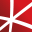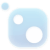# Top 20 NuGet statistics Packages

Math.NET Numerics is the numerical foundation of the Math.NET project, aiming to provide methods and algorithms for numerical computations in science, engineering and every day use. Supports .NET 5.0 or higher, .NET Standard 2.0 and .NET Framework 4.6.1 or higher, on Windows, Linux and Mac. This pac...
Math.NET Numerics is the numerical foundation of the Math.NET project, aiming to provide methods and algorithms for numerical computations in science, engineering and every day use. Supports .NET 5.0 or higher, .NET Standard 2.0 and .NET Framework 4.6.1 or higher, on Windows, Linux and Mac.
F# Modules for Math.NET Numerics, the numerical foundation of the Math.NET project, aiming to provide methods and algorithms for numerical computations in science, engineering and every day use. Supports .NET 5.0 or higher, .NET Standard 2.0 and .NET Framework 4.6.1 or higher, on Windows, Linux and ...## NMath Stats Premium - Microsoft Chart Controls

Charting for CenterSpace.NMath.Stats.Premium types using the Microsoft Chart Controls for .NET, creating a complete data analysis and visualization solution.
The GPU-accelerated version of package CenterSpace.NMath.Stats. With a few minor exceptions, such as optional GPU configuration settings, the API is identical between CenterSpace.NMath.Stats.Premium and CenterSpace.NMath.Stats.## NMath Stats - Microsoft Chart Controls

Charting for CenterSpace.NMath.Stats types using the Microsoft Chart Controls for .NET, creating a complete data analysis and visualization solution.
Classes for data manipulation and statistical computation, including descriptive statistics, probability distributions, combinatorial functions, multiple linear regression, hypothesis testing, analysis of variance, multivariate statistics, partial least squares, and nonnegative matrix factorization.
Contains probability distributions, statistical models and methods such as Linear and Logistic regression, Hidden Markov Models, (Hidden) Conditional Random Fields, Principal Component Analysis, Partial Least Squares, Discriminant Analysis, Kernel methods and functions and many other related techniq...
Foundational classes for financial, engineering, and scientific applications, including complex number classes, general vector and matrix classes, structured sparse matrix classes and factorizations, general sparse matrix classes and factorizations, general matrix decompositions, least squares solut...
The GPU-accelerated version of package CenterSpace.NMath. With a few minor exceptions, such as optional GPU configuration settings, the API is identical between CenterSpace.NMath.Premium and CenterSpace.NMath. If using at least .NET Framework 4.6.1 or .NET Core 2.0, we recommend using one of our NMa...
The Extreme Optimization Numerical Libraries for .NET are a set of libraries for numerical computing and data analysis. This is the main package that contains all the core functionality. For optimal performance, we strongly recommend also referencing one of the native packages based on Intel's Mat...## Math.NET Numerics - MKL Native Provider for Windows (x64)

Intel oneAPI MKL native libraries for Math.NET Numerics on Windows.
Deedle implements an efficient and robust frame and series data structures for manipulating with structured data. It supports handling of missing values, aggregations, grouping, joining, statistical functions and more. For frames and series with ordered indices (such as time series), automatic align...
F# Modules for Math.NET Numerics, the numerical foundation of the Math.NET project, aiming to provide methods and algorithms for numerical computations in science, engineering and every day use. Supports .NET 5.0 or higher, .NET Standard 2.0 and .NET Framework 4.6.1 or higher, on Windows, Linux and ...
Foundational classes for financial, engineering, and scientific applications, including complex number classes, general vector and matrix classes, structured sparse matrix classes and factorizations, general sparse matrix classes and factorizations, general matrix decompositions, least squares solut...## Math.NET Numerics - MKL Native Provider for Windows (x64 and x86)

Intel oneAPI MKL native libraries for Math.NET Numerics on Windows.
StatsN.Contracts## Math.NET Numerics - MKL Native Provider for Windows (x86)

Intel oneAPI MKL native libraries for Math.NET Numerics on Windows.
Supports various mathematical and numerical methods.